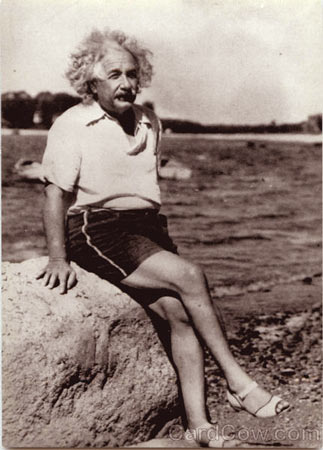A FINAL ARGUMENT AGAINST
x’ = x – vt

by Miles MathisNice Shoes!

In my long paper on Special Relativity I offer several strong arguments against this equation at the beginning of my proof. However I recently discovered another strong argument. It is the subject of this brief addendum.
I was re-reading Richard Feynman’s Six Not-So-Easy Pieces (a book I have attacked in several of my papers, including the Michelson paper and the a = v2/r paper), and found that he was using the equation in his lectures.
This book was first published in 1997: 92 years after Einstein’s paper. Feynman diagrams Special Relativity precisely the way Einstein did. His illustration matches the illustration I published with my proof point for point. So does his first equation: x’ = x – vt [see p.51]. This is Einstein’s first equation, both in the paper of 1905 and in the book Relativity. Feynman accepts it without question.
Let x’ be a distance in a moving coordinate system; x is the same distance measured from a stationary system. Feynman then explains it this way: “After time t the origin [of x’] has moved a distance vt, and if the two origins originally coincided, then x’ = x – vt.”
Feynman also confirms what Einstein told us in 1905: this equation is the classical transformation from one system to the other. Einstein calls it a Galilei transformation and Feynman calls it Newton’s principle of relativity, but the implication is the same. It is just the accepted equation. It is supposed to be what the Lorentz transformations reduce to if the speed of light is infinite.
The equation is telling us that vt is the distance between the two coordinate systems, and that you add this distance to x’ to get x. Feynman confirms this. It could not be more straightforward.
But the origin of x’ is not moving. If the origins of the two coordinate systems were together at t0 , then they are still together, since origins don’t move, by definition. This is just to say that if the train started from the station at t0 , then after time t the train still started from the station, which has not moved. Train stations do not move, just as origins do not move: t0’ and x0’ are still back at the origin, which is still back at the train station.
Einstein and Feynman are mistaking the back end of the caboose with the origin. Look at what the equation is telling us. Let’s say at t0 the back end of the caboose is at the origin of the moving system, S’. Let’s also say that x’ is the distance to the front of the same caboose, as measured from inside the caboose. The whole train then leaves us at the station and travels a distance given by the term vt. The equation x = x’ + vt is telling us that we, back at the station, will measure the length of the caboose as “how long the caboose is, measured from the caboose” + “the distance it has gone”. As if we will add the length of the traintracks to the length of the caboose! Do you see now how utterly absurd this is? It assumes that we can’t see, with our own eyes, that the back of the caboose has also traveled vt, and must therefore be subtracted from x’ + vt. What we are looking for in this problem is simply “how long the caboose looks to us.” This equation tells us nothing about that at all, not classically, not relativistically, nothing. It is the wrong equation. Classically, the correct equation is just Δx’ = Δx, as I have shown exhaustively elsewhere. Einstein imported an equation that Newton would have used to find the total distance from the origin to the front of the caboose after time t, and applied it to find the length of the caboose as seen from the origin. An absolutely momentous blunder, magnified by its being missed by a thousand Feynmans.
If light had an infinite speed, then the embankment would see both ends of any rod at the same time. The embankment would also see the origin and the point at the same time. If c is infinite, then all measurers will measure equal times, distances and velocities, in all directions and on all possible axes. x’ = x, v’ = v. This is because there is no difference between what I see and what the train sees. Light brings me exactly the same information that it brings the train, at exactly the same time. There can be no transformation equation: not a fancy Lorentz transformation, but also not a simple transformation like x’ = x - vt . So the given equation is not a Galilei transformation, in any sense.

go to homepage
go to chapter 10

If this paper was useful to you in any way, please consider donating a dollar (or more) to the SAVE THE ARTISTS FOUNDATION. This will allow me to continue writing these "unpublishable" things. Don't be confused by paying Melisa Smith--that is just one of my many noms de plume. If you are a Paypal user, there is no fee; so it might be worth your while to become one. Otherwise they will rob us 33 cents for each transaction.